xGeostrophic windOverview

The geostrophic wind is the theoretical wind
Wind
Wind is the flow of gases on a large scale. On Earth, wind consists of the bulk movement of air. In outer space, solar wind is the movement of gases or charged particles from the sun through space, while planetary wind is the outgassing of light chemical elements from a planet's atmosphere into space...

that would result from an exact balance between the Coriolis effect
Coriolis effect
In physics, the Coriolis effect is a deflection of moving objects when they are viewed in a rotating reference frame. In a reference frame with clockwise rotation, the deflection is to the left of the motion of the object; in one with counter-clockwise rotation, the deflection is to the right...

and the pressure gradient
Pressure gradient
In atmospheric sciences , the pressure gradient is a physical quantity that describes in which direction and at what rate the pressure changes the most rapidly around a particular location. The pressure gradient is a dimensional quantity expressed in units of pressure per unit length...

force. This condition is called geostrophic balance. The geostrophic wind is directed parallel
Parallel (geometry)
Parallelism is a term in geometry and in everyday life that refers to a property in Euclidean space of two or more lines or planes, or a combination of these. The assumed existence and properties of parallel lines are the basis of Euclid's parallel postulate. Two lines in a plane that do not...

to isobars (lines of constant pressure
Atmospheric pressure
Atmospheric pressure is the force per unit area exerted into a surface by the weight of air above that surface in the atmosphere of Earth . In most circumstances atmospheric pressure is closely approximated by the hydrostatic pressure caused by the weight of air above the measurement point...

at a given height). This balance seldom holds exactly in nature. The true wind almost always differs from the geostrophic wind due to other forces such as friction
Friction
Friction is the force resisting the relative motion of solid surfaces, fluid layers, and/or material elements sliding against each other. There are several types of friction:...

from the ground.Unanswered QuestionsWhy geostrophic wind not experienced at equatorEncyclopedia
The geostrophic wind is the theoretical wind
Wind
Wind is the flow of gases on a large scale. On Earth, wind consists of the bulk movement of air. In outer space, solar wind is the movement of gases or charged particles from the sun through space, while planetary wind is the outgassing of light chemical elements from a planet's atmosphere into space...

that would result from an exact balance between the Coriolis effect
Coriolis effect
In physics, the Coriolis effect is a deflection of moving objects when they are viewed in a rotating reference frame. In a reference frame with clockwise rotation, the deflection is to the left of the motion of the object; in one with counter-clockwise rotation, the deflection is to the right...

and the pressure gradient
Pressure gradient
In atmospheric sciences , the pressure gradient is a physical quantity that describes in which direction and at what rate the pressure changes the most rapidly around a particular location. The pressure gradient is a dimensional quantity expressed in units of pressure per unit length...

force. This condition is called geostrophic balance. The geostrophic wind is directed parallel
Parallel (geometry)
Parallelism is a term in geometry and in everyday life that refers to a property in Euclidean space of two or more lines or planes, or a combination of these. The assumed existence and properties of parallel lines are the basis of Euclid's parallel postulate. Two lines in a plane that do not...

to isobars (lines of constant pressure
Atmospheric pressure
Atmospheric pressure is the force per unit area exerted into a surface by the weight of air above that surface in the atmosphere of Earth . In most circumstances atmospheric pressure is closely approximated by the hydrostatic pressure caused by the weight of air above the measurement point...

at a given height). This balance seldom holds exactly in nature. The true wind almost always differs from the geostrophic wind due to other forces such as friction
Friction
Friction is the force resisting the relative motion of solid surfaces, fluid layers, and/or material elements sliding against each other. There are several types of friction:...

from the ground. Thus, the actual wind would equal the geostrophic wind only if there were no friction and the isobars were perfectly straight. Despite this, much of the atmosphere outside the tropics
Tropics
The tropics is a region of the Earth surrounding the Equator. It is limited in latitude by the Tropic of Cancer in the northern hemisphere at approximately  N and the Tropic of Capricorn in the southern hemisphere at  S; these latitudes correspond to the axial tilt of the Earth...

is close to geostrophic flow much of the time and it is a valuable first approximation. Geostrophic flow in air or water is a zero-frequency inertial wave.

## Origin

Air naturally moves from areas of high pressure
Pressure
Pressure is the force per unit area applied in a direction perpendicular to the surface of an object. Gauge pressure is the pressure relative to the local atmospheric or ambient pressure.- Definition :...

to areas of low pressure, due to the pressure gradient
Pressure gradient
In atmospheric sciences , the pressure gradient is a physical quantity that describes in which direction and at what rate the pressure changes the most rapidly around a particular location. The pressure gradient is a dimensional quantity expressed in units of pressure per unit length...

force. As soon as the air starts to move, however, the Coriolis "force"
Coriolis effect
In physics, the Coriolis effect is a deflection of moving objects when they are viewed in a rotating reference frame. In a reference frame with clockwise rotation, the deflection is to the left of the motion of the object; in one with counter-clockwise rotation, the deflection is to the right...

deflects it. The is to the right in the northern hemisphere
Northern Hemisphere
The Northern Hemisphere is the half of a planet that is north of its equator—the word hemisphere literally means “half sphere”. It is also that half of the celestial sphere north of the celestial equator...

, and to the left in the southern hemisphere
Southern Hemisphere
The Southern Hemisphere is the part of Earth that lies south of the equator. The word hemisphere literally means 'half ball' or "half sphere"...

. As the air moves from the high pressure area, its speed increases, and so does its Coriolis deflection. The deflection increases until the Coriolis and pressure gradient forces are in geostrophic balance: at this point, the air flow is no longer moving from high to low pressure, but instead moves along an . (Note that this explanation assumes that the atmosphere starts in a geostrophically unbalanced state and describes how such a state would evolve into a balanced flow. In practice, the flow is nearly always balanced.) The geostrophic balance helps to explain why, in the northern hemisphere, low pressure systems (or cyclone
Cyclone
In meteorology, a cyclone is an area of closed, circular fluid motion rotating in the same direction as the Earth. This is usually characterized by inward spiraling winds that rotate anticlockwise in the Northern Hemisphere and clockwise in the Southern Hemisphere of the Earth. Most large-scale...

s
) spin counterclockwise and high pressure systems
High pressure area
A high-pressure area is a region where the atmospheric pressure at the surface of the planet is greater than its surrounding environment. Winds within high-pressure areas flow outward due to the higher density air near their center and friction with land...

(or anticyclone
Anticyclone
An anticyclone is a weather phenomenon defined by the United States' National Weather Service's glossary as "[a] large-scale circulation of winds around a central region of high atmospheric pressure, clockwise in the Northern Hemisphere, counterclockwise in the Southern Hemisphere"...

s
) spin clockwise, and the opposite in the southern hemisphere.

## Geostrophic currents

Flow of ocean water is also largely geostrophic. Just as multiple weather balloons that measure pressure as a function of height in the atmosphere are used to map the atmospheric pressure field and infer the geostrophic wind, measurements of density as a function of depth in the ocean are used to infer geostrophic currents. Satellite altimeters are also used to measure sea surface height anomaly, which permits a calculation of the geostrophic current at the surface.

## Limitations of the Geostrophic approximation

The effect of friction, between the air and the land, breaks the geostrophic balance. Friction slows the flow, lessening the effect of the Coriolis force. As a result, the pressure gradient force has a greater effect and the air still moves from high pressure to low pressure, though with great deflection. This explains why high pressure system winds radiate out from the center of the system, while low pressure systems have winds that spiral inwards.

The geostrophic wind neglects friction
Friction
Friction is the force resisting the relative motion of solid surfaces, fluid layers, and/or material elements sliding against each other. There are several types of friction:...

al effects, which is usually a good approximation
Approximation
An approximation is a representation of something that is not exact, but still close enough to be useful. Although approximation is most often applied to numbers, it is also frequently applied to such things as mathematical functions, shapes, and physical laws.Approximations may be used because...

for the synoptic scale
Synoptic scale meteorology
The synoptic scale in meteorology is a horizontal length scale of the order of 1000 kilometres or more. This corresponds to a horizontal scale typical of mid-latitude depressions...

instantaneous flow in the midlatitude mid-troposphere
Troposphere
The troposphere is the lowest portion of Earth's atmosphere. It contains approximately 80% of the atmosphere's mass and 99% of its water vapor and aerosols....

. Although ageostrophic terms are relatively small, they are essential for the time evolution of the flow and in particular are necessary for the growth and decay of storms. Quasigeostrophic and Semigeostrophic theory are used to model flows in the atmosphere more widely. These theories allow for divergence to take place and for weather systems to then develop.

## Governing formula

Newton's Second law can be written as follows if only the pressure gradient, gravity, and friction act on an air parcel, where the bold symbolizes a vector.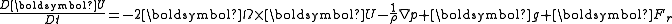This can be expanded in spherical coordinates, with a positive u representing an eastward direction and a positive v representing a northward direction.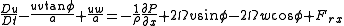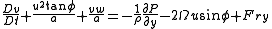For some systems such as midlatitude cyclones, the only two terms which contribute significantly to the horizontal momentum equations are the Coriolis and pressure gradient terms.

Assuming geostrophic balance, the geostrophic wind components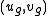on a constant-pressure surface can be derived as: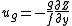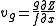where g is the acceleration due to gravity
Standard gravity
Standard gravity, or standard acceleration due to free fall, usually denoted by g0 or gn, is the nominal acceleration of an object in a vacuum near the surface of the Earth. It is defined as precisely , or about...

(9.81 m.s−2), f is the Coriolis parameter
Coriolis effect
In physics, the Coriolis effect is a deflection of moving objects when they are viewed in a rotating reference frame. In a reference frame with clockwise rotation, the deflection is to the left of the motion of the object; in one with counter-clockwise rotation, the deflection is to the right...

(approximately 10−4 s−1, varying with latitude) and Z is the geopotential height
Geopotential height
Geopotential height is a vertical coordinate referenced to Earth's mean sea level — an adjustment to geometric height using the variation of gravity with latitude and elevation. Thus it can be considered a "gravity-adjusted height"...

of the constant pressure surface. The validity of this approximation depends on the local Rossby number
Rossby number
The Rossby number, named for Carl-Gustav Arvid Rossby, is a dimensionless number used in describing fluid flow. The Rossby number is the ratio of inertial to Coriolis force, terms v\cdot\nabla v\sim U^2 / L and \Omega\times v\sim U\Omega in the Navier–Stokes equations, respectively...

. It is invalid at the equator, because f is equal to zero there, and therefore generally not used in the tropics
Tropics
The tropics is a region of the Earth surrounding the Equator. It is limited in latitude by the Tropic of Cancer in the northern hemisphere at approximately  N and the Tropic of Capricorn in the southern hemisphere at  S; these latitudes correspond to the axial tilt of the Earth...

.

Other variants of the equation are possible; for example, the geostrophic wind vector can be expressed in terms of the gradient of the geopotential height
Geopotential height
Geopotential height is a vertical coordinate referenced to Earth's mean sea level — an adjustment to geometric height using the variation of gravity with latitude and elevation. Thus it can be considered a "gravity-adjusted height"...

Φ on a surface of constant pressure: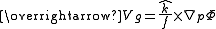## External links

The source of this article is wikipedia, the free encyclopedia.  The text of this article is licensed under the GFDL.